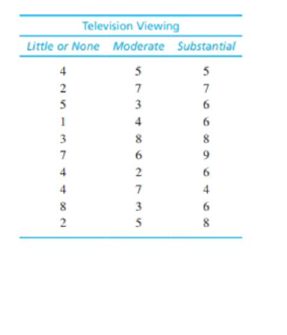Chapter 12, Problem 24PEssentials of Statistics for the B...

8th Edition
Frederick J Gravetter + 1 other
ISBN: 9781133956570

Solutions

Chapter
SectionEssentials of Statistics for the B...

8th Edition
Frederick J Gravetter + 1 other
ISBN: 9781133956570
Textbook Problem

New research suggests that watching television, especially medical shows such as Grey’s Anatomy and House, can result in increased concern about personal health (Ye, 2010). Surveys administered to college students measure television viewing habits and health concerns such as fear of developing the diseases and disorders seen on television. For the following data students are classified into three categories based on their television viewing patterns and health concerns are measured on a 10-point scale with 0 indicating “none."a. Use an ANOVA with α = .05 to determine whether there are significant mean differences among the three groups.b. Compute η2 to measure the size of the effect.c. Use Tukey's HSD test with α = .05 to determine which groups are significantly different.

a.

To determine

To Find: Whether there is any significant difference among the three groups using ANOVA with α=0.05

Explanation

Given info: One survey was done on students on watching television of medical shows. The students were classified into three categories based on their television viewing patterns and health concerns on 10 point scale.

Calculations:

The null and alternative hypotheses:

Null hypothesis:

H0:μ1=μ2=μ3

That is, all treatments have equal mean.

Alternate hypothesis:

Ha:at least one of the treatments is different.

Degrees of freedom:

It is known that for k number of treatments the degrees of freedom is,

df=k1.

Thus,

dfbetween=k1=2

Similarly,

dfwithin=Nk=303=27

Critical value:

For α=0.05 and df=(2,27) the critical value is 3

b.

To determine

To Find: The η2 for the given question.

c.

To determine

To Find: Which groups are significantly different using Tukey’s HSD.

Still sussing out bartleby?

Check out a sample textbook solution.

See a sample solution

The Solution to Your Study Problems

Bartleby provides explanations to thousands of textbook problems written by our experts, many with advanced degrees!

Get Started

Find the limit: limx3x2+x12x3

Calculus: An Applied Approach (MindTap Course List)

Evaluate dy if y = x3 2x2 + 1, x = 2, and dx = 0.2.

Single Variable Calculus: Early Transcendentals

In Exercises 516, evaluate the given quantity. log100,000

Finite Mathematics and Applied Calculus (MindTap Course List)

Graph each of the following lines. y=2x

Trigonometry (MindTap Course List)